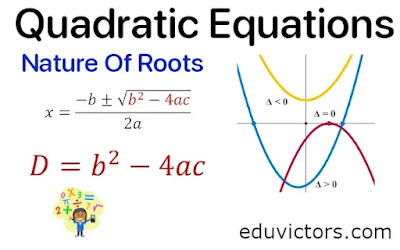# CBSE Class 10 Maths - Quadratic Equations - Nature of Roots (Solved Problems)

We know that A polynomial p(x) = ax² + bx + c of degree 2 is called a quadratic polynomial, then p(x) = 0 is known as quadratic equation.

There are three methods to find the solution of a quadratic equation:

1. Factorisation method

2. Method of completing the square

What is a Discriminant of Quadratic Equation ax² + bc + c = 0 ?

In general, Discriminant is represented by symbol D.

Mathematically,

D = $D = b^2 - 4ac$

The Discriminant helps us find the nature of roots.

If D > 0 i.e. $b^2 - 4ac > 0$ then the given quadratic equation (QE) has two distinct real roots. (Note: Distinct mean unique values, real mean real numbers).

Value of roots will be :

$x = \frac{-b + \sqrt{D}}{2a}$ and $x = \frac{-b - \sqrt{D}}{2a}$

If D = 0 i.e. $b^2 - 4ac > 0$ then the QE has equal real roots.

i.e. $x = \frac{-b}{2a}$ and $x = \frac{-b}{2a}$

If D < 0 then $\sqrt{D} = \sqrt{-ve }$, there is no real roots exist.

We can say roots are imaginary or complex numbers (that you'll study in Class XI).

Sum of Roots of a quadratic equation:

For real roots, α = $\frac{-b + \sqrt{D}}{2a}$ and β = $\frac{-b - \sqrt{D}}{2a}$

Now α + β = \frac{-b + \sqrt{D}}{2a} +\frac{-b - \sqrt{D}}{2a}$=$\frac{-b + \sqrt{D} -b - \sqrt{D}}{2a} $=$\frac{-2b}{2a} $=$\frac{-b}{a}$Product of roots of a quadratic equation: For real roots, α =$\frac{-b + \sqrt{D}}{2a}$and β =$\frac{-b - \sqrt{D}}{2a}$Now α × β =$\frac{-b + \sqrt{D}}{2a} \times \frac{-b - \sqrt{D}}{2a} $=$\frac{(-b)^2 - (\sqrt{D})^2}{4a^2} $=$\frac{b^2 - D}{4a^2} $=$\frac{b^2 -b^2 + 4ac}{4a^2}$=$\frac{4ac}{4a^2}$=$\frac{c}{a}$For a quadratic equation ax² + bx + c = 0, If sum of roots is 0, then b = 0 If roots are reciprocal of each other then a = c If products of roots is 0, then c = 0. Q1: Write the discriminant of each of the following quadratic equations. Find the nature of roots also. x² + 4x + 3 =0 Answer: D = b² - 4ac In the given QE, a = 1, b = 4, c = 3 ∴ D = 4² - 4(1)(3) D = 16 - 12 D = 4 ∵ D > 0, roots are distinct and real. Q2: Write the discriminant of each of the following quadratic equation: 2x² + 4x + 5 = 0 Answer: In the given QE, a = 2, b = 4 and c = 5 D = b² - 4ac ∴ D = 4² - 4(2)(5) D = 16 - 40 D = -24 ∵ D < 0, roots are non-real or imaginary. Q3: Write the discriminant of each of the following quadratic equations and also find the nature of roots.$\sqrt{3}x^2 -2\sqrt{2} - 2\sqrt{3} = 0$Answer: In the given quadratic equation, a =$\sqrt{3}$, b =$-2\sqrt{2}$and c =$- 2\sqrt{3}$D = b² - 4ac ∴ D =$(-2\sqrt{2})^2 - 4(\sqrt{3})(- 2\sqrt{3})\$

= 8 - (-24)

= 8 + 24

= 32

∵ D > 0, roots are distinct and real.

Q4: Write the discriminant of each of the following quadratic equations and also find the nature of roots.

x² + 6x + 9 = 0

In the given QE, a = 1, b = 6 and c = 9

D = b² - 4ac

∴ D = 6² - 4(1)(9)

D = 36 - 36

D = 0

∵ D = 0, roots are real and equal.

Q5: Find the value of k, so that the quadratic equation

(k + 1) x² – 2 (k — 1) x + 1 = 0 has equal roots.

a = k + 1, b = -2(k - 1) and c = 1

For equal roots Disrciminant D = 0

∴  D = b² - 4ac = 0

⇒ (-2(k - 1))² - 4(k + 1)(1) = 0

4(k-1)² - 4k - 4 = 0

4[(k-1)² -k - 1] = 0

4[k² -2k + 1 -k -1] = 0

4[k² -3k] = 0

⇒ 4k(k-3) = 0

⇒ 4 ≠ 0, k = 0 or k - 3 = 0

⇒ k = 0 or k = 3

∴ Values of k are 0, 3